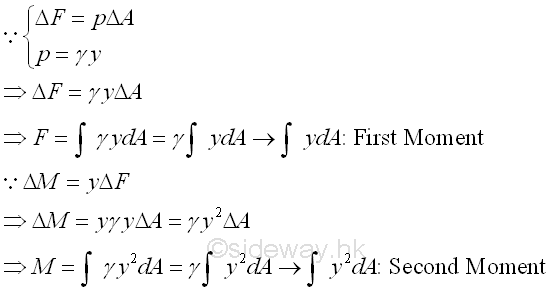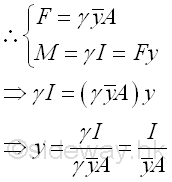output.to from Sideway
Mechanics: Statics

Draft for Information Only

# Content

`Second Moment Moment of Inertia`

# Second Moment

Moment is the concept of a concentrated force about a point and is proportional to the distance between the force and the reference point.  In general, the first moment of a body about a reference is the summation of the moment of uniformly distributed forces over the body relating to the first order of distance and the shape of the body only. Although, the moment of distributed forces of intensity proportional to the distance between the force and the reference is also proportional to the distance between the force and the reference, the summation of the moment of the distributed forces  of intensity proportional to the distance over the body relating to the square of the distance or the second order of distance and the shape of the body. Since these are common terms found in practical calculation, they are defined as the property of a body and is called the second moment of a body. For example, force acting on submerged plane surface can be expressed in terms of the differential elemental area, ΔA and the pressure, a function of the depth y, acting on the element. ImplySince the elemental force or the distributed forces is a function the depth y, the resultant force F can be determined by making use of the first moment of the body. Similarly, since the elemental moment of the distruibuted forces about a reference is a function of the square of the depth y,  the resultant moment M can be determined by making use of the second moment of the body. And the point of application y of the resultant force F can be determined through system of forces transformation by equating the moment of the two system of forces. Imply## Moment of Inertia

The second moment of an area is also called moment of inertia or area moment of inertia. Besides, the application of fluid pressure of nonhorizontal submerged plane surface, second moment of an area is also involved in many engineering application analysis, e.g. bending stress, shearing stress, torsional shearing stress, angle of twist, buckling of a column, the area moment of inertia is usually refered to an plane area about an axis either parallel or normal to the plane area as the property of an plane area.

Moment of inertia is commonly used for both areas and masses.  The second moment of a mass is usually used to  measure a body of mass to resist changes in rotational acceleration about an axis. The tendency to resist changes in their state of motion is called as inertia. The tendency to resist rotational acceleration obtained by the second moment of a mass is therefore called the moment of inertia of the body mass about the axis or the mass moment of inertia or moment of inertia.

©sidewayReferences

1. I.C. Jong; B.G. rogers, 1991, Engineering Mechanics: Statics and Dynamics, Saunders College Publishing, United States of America
2. F.P. Beer; E.R. Johnston,Jr.; E.R. Eisenberg, 2004, Vector Mechanics for Engineers: Statics, McGraw-Hill Companies, Inc., New YorkID: 121000001 Last Updated: 2013/8/17 Revision: 2 Ref:Home (5)

Business

Management

HBR (3)

Information

Recreation

Hobbies (7)

Culture

Chinese (1097)

English (336)

Reference (66)

Computer

Hardware (149)

Software

Application (187)

Digitization (24)

Numeric (19)

Programming

Web (644)CSS (SC)

ASP.NET (SC)

HTML

Knowledge Base

Common Color (SC)

Html 401 Special (SC)

OS (389)MS Windows

Windows10 (SC)

.NET Framework (SC)

DeskTop (7)

Knowledge

Mathematics

Formulas (8)

Number Theory (206)

Algebra (20)

Trigonometry (18)

Geometry (18)

Calculus (67)

Complex Analysis (21)

Engineering

Tables (8)

Mechanical

Mechanics (1)

Rigid Bodies

Statics (92)

Dynamics (37)

Fluid (5)

Control

Acoustics (19)

Biology (1)

Geography (1)

Latest Updated Links

Copyright © 2000-2019 Sideway . All rights reserved Disclaimers last modified on 10 Feb 2019